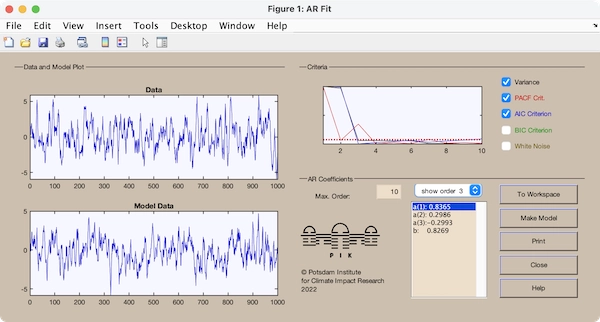# CRP Toolbox

## arfit

AR parameter estimation via Yule-Walker method.

### Syntax

``````arfit(x,p)
a=arfit(x,p)
[a y]=arfit(x,p)
``````

### Description

arfit(x,p) opens a GUI for AR coefficients estimation for the vector x using the Yule-Walker method. The coefficients and order selection criterias for all orders until the maximal order p will be solved. The coefficients are solved by the Levinson-Durbin algorithmus. The criteria are normalized in order to show them in the same plot.

a=arfit(x,p) returns the vector a of length (p+1) of the AR coefficients and the noise level for the corresponding AR model of the model order p. The GUI is suppressed.

[a y]=arfit(x,p) returns the vector y of a realization of the resulting AR model. The GUI is suppressed.

### Examples

``````x=rand(3,1);
a=[.8 .3 -.25 .9]';
for i=4:1000,
x(i)=sum(a(1:3).*x(i-1:-1:i-3))+a(end)*randn;
end
arfit(x,10)
``````